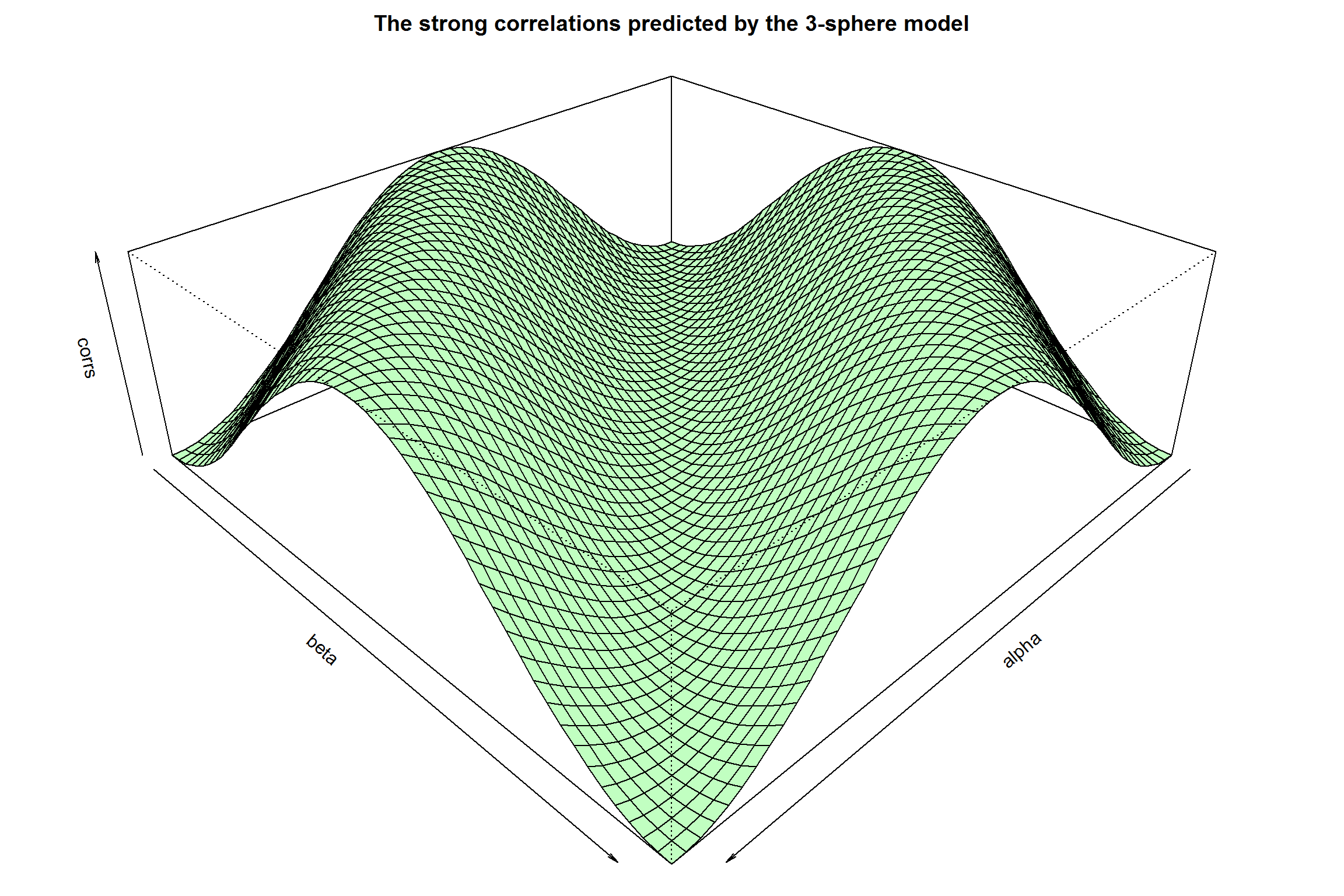# There are no zero outcomes in the S^3 model for EPR-B

by Joy Christian

Einstein Centre for Local-Realistic Physics, 15 Thackley End, Oxford OX2 6LB, United Kingdom

Publication date: 24 August 2015

# For a theoretical background, please see http://arxiv.org/abs/1405.2355

# and http://www.sciphysicsforums.com/spfbb1/viewtopic.php?f=6&t=188#p5129
Angles = seq(from = 0, to = 360, by = 7.2) * 2 * pi/360

K = length(Angles) # The total number of angles between 0 and 2pi

corrs = matrix(nrow = K, ncol = K, data = 0) # Container for correlations

Ns  = matrix(nrow = K, ncol = K, data = 0) # Container for initial states
M = 10^4 # Size of the pre-ensemble. Next one can try 10^5, or even 10^6

r = runif(M, 0, 2*pi) # M uniformly distributed numbers between 0 and 2pi

z = runif(M, -1, +1) # M uniformly distributed numbers between -1 and +1

h = sqrt(1 - z^2)

x = h * cos(r)

y = h * sin(r)

w = rbind(x, y, z)  # A 3xM matrix, with M columns of w-vectors representing
# the x, y, z coordinates of uniformly distributed points on an S^2. In what
# follows these M number of pre-states will define an auxiliary pre-ensemble
s = runif(M, 0, pi) # Initial states of the spin are the pairs (w,s) within S^3

f = -1 + (2/sqrt(1 + ((3 * s)/pi))) # For details see the paper arXiv:1405.2355

g = function(u,v,s){ifelse(abs(colSums(u*v)) > f, colSums(u*v), 0)}
for (i in 1:K) {
alpha = Angles[i]
a = c(cos(alpha), sin(alpha), 0)  # Measurement direction 'a'

for (j in 1:K) {
beta = Angles[j]
b = c(cos(beta), sin(beta), 0)  # Measurement direction 'b'

# Event-ready preparation of the initial states e

C = sign(g(a,w,s))
D = sign(g(b,w,s))

o = x[C & D]
p = y[C & D]
q = z[C & D]

e = rbind(o,p,q) # The event-readied initial states of spin

N = length(e)/3 # The total number of the initial states e

# Computing the "quantum" correlation

a.e = colSums(a*e) # dot product between the vectors a and e
b.e = colSums(b*e) # dot product between the vectors b and e

A = +sign(a.e)  # Alice's measurement results A(a,e) = +/-1

B = -sign(b.e)  # Bob's measurement results B(b, e) = +/-1

corrs[i,j] = sum(A*B)/N # The correlation coefficient E(a,b)

# corrs[i,j] = length(A*B)/N # Verifies (# of A*B) = (# of e)

# corrs[i] = sum(A)/N # Verifies < A > = 0 for all vectors a

# corrs[j] = sum(B)/N # Verifies < B > = 0 for all vectors b

# Plotting the "quantum" correlation

Ns[i,j] = N # The total number of initial states in the graph
}
}
par(mar = c(0, 0, 2, 0))
persp(x = Angles, y = Angles, main = "The strong correlations predicted by the 3-sphere model", z = corrs, zlim = c(-1, 1), col = "darkseagreen1", theta = 135, phi = 30, scale = FALSE, xlab = "alpha", ylab = "beta")